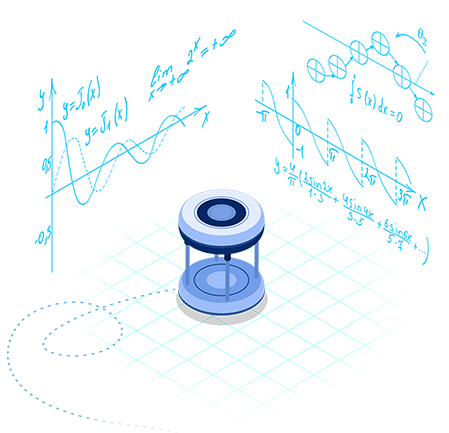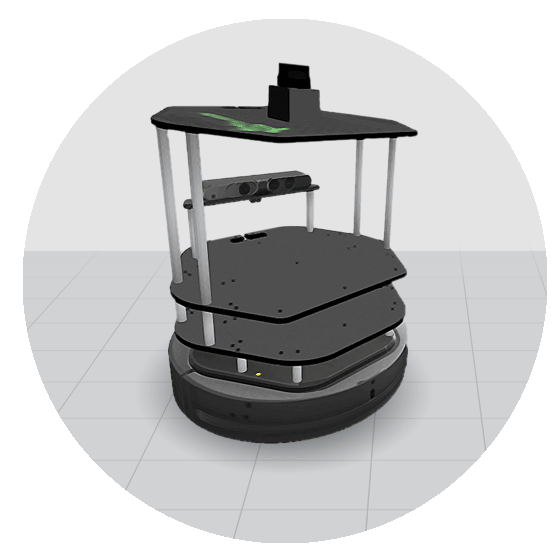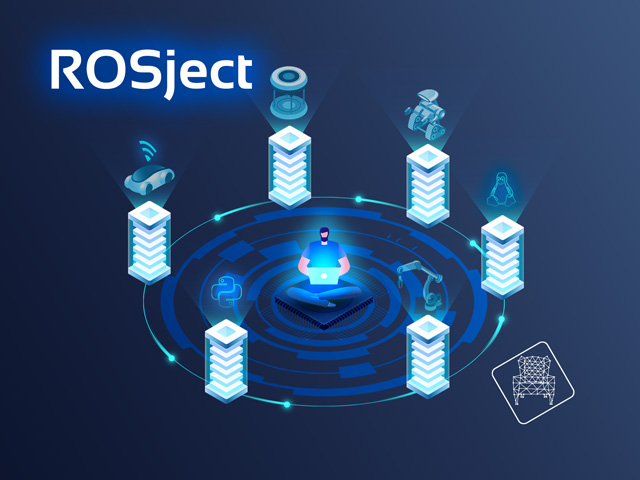# Basic Maths for Robotics Course - Python

Learn the most useful Mathematics: the ones we can apply to Robotics!## Course Summary

Mathematics are the key to describe everything we can appreciate or interact with in nature. Thus, a robot also needs the abilities to move its mechanisms (locomotion), sense the environment (perception), reason accordingly (cognition), and take actions in the environment (navigation). A good understanding of what geometry stands for, basic linear algebra, calculus and operations with numbers, and some probability theory will be necessary to become a robotics developer.

## What you will learn

• Linear Algebra, where you'll learn about vectors and matrices

• Calculus, where you'll learn about functions, derivatives, and integrals

• Probability, where you'll learn about random variables and belief distributions

## Course Overview

### Introduction

Unit for previewing the contents of the Course.

### Linear Algebra (vectors and matrices)

In this chapter, you are going to get a gentle introduction to the most basic field of mathematics: Linear Algebra. This discipline is a prerequisite to any career you want to follow in mathematics, physics, engineering, etc.

### Calculus

In this chapter, you are going to be introduced to the basic unit of calculus: a function. In mathematics, this unit provides valuable information on how a variable changes. It may change over time, position, or orientation, but it all can be studied with the properties of functions.

### Probability

Autonomous robots develop their behaviours in pairs of perception of their state and the environment, and action from their motors to the environment. Probability is a representation of how uncertain we can be that the robot perceives what we think, or performs the actions we want.

### Project: Escape of a maze

In the final project of this course, you are going to help a mobile robot escape of a maze. It consists of making a program that dictates the robot the movements to perform in order to get out of the maze.

## Teachers

### Irene Pérez

My name is Irene, I'm a Physicist and Engineer from Barcelona. I'm passionate about applying AI to Robotics, while focusing in the utility and beauty of fundamental mathematics and physics involved. ROS gives me the tools to enjoy all of that!## Robots used

### Turtlebot robot## Learning Path

Basic Robotics Theory